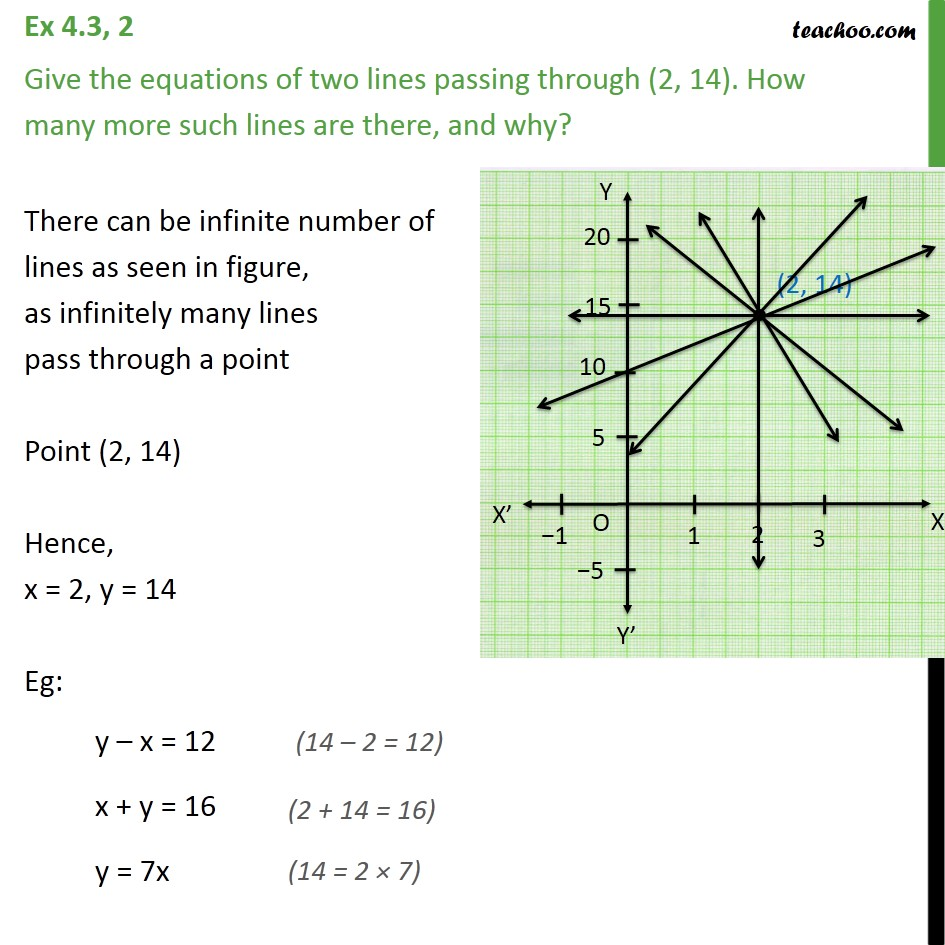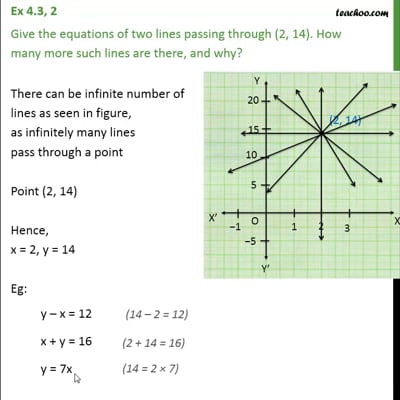Ex 4.3

Chapter 4 Class 9 Linear Equations in Two Variables
Serial order wiseThis video is only available for Teachoo black users

Maths Crash Course - Live lectures + all videos + Real time Doubt solving!

### Transcript

Ex 4.3, 2 Give the equations of two lines passing through (2, 14). How many more such lines are there, and why? There can be infinite number of lines as seen in figure, as infinitely many lines pass through a point Point (2, 14) Hence, x = 2, y = 14 Eg: y – x = 12 x + y = 16 y = 7x